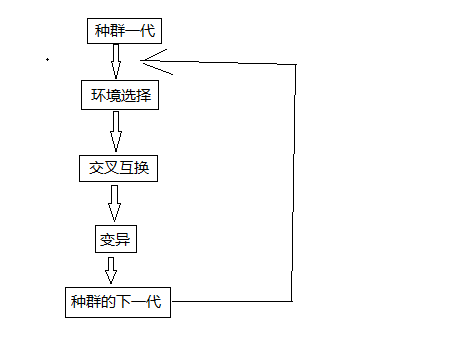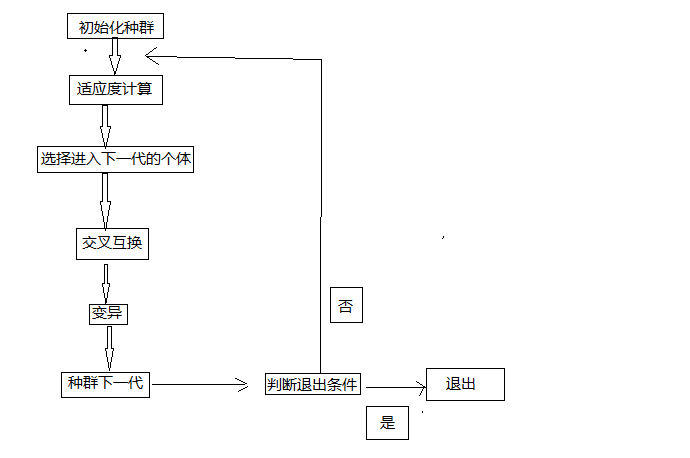• 遗传算法的应用实例
2022-03-28 14:37:10

### 二进制编码为基础

%目标函数
function y = fobj(x)
if x <= 0
y = sin(x);
elseif x <= 2
y = x^2/2;
else
y = 3-x/2;
end
end

%主程序
%清楚窗口内容和变量
clear
clc

pc = 0.8 ;%交叉率
pm = 0.05 ;%变异率

Iter_N =100; %迭代最大代数
popsize = 10; %种群规模，个体的集合

LB = -10;
UB = 10;  %左右边界
DELTA = 1e-2;
x=LB:0.05:UB;

for i = 1:length(x)
y(i)=fobj(x(i));  %目标函数
end
plot(x,y)
hold on;

1. 初始化种群
function pop = ini_pop(LB,UB,DELTA,popsize)
N = ceil(log2((UB-LB)/DELTA+1)); %
for i = 1:popsize
pop(i,:)=randi(2,[1,N])-1; %
end


%2. fitness 计算适应性
function y = fitness(LB,UB,x)
popsize = size(x,1);
for i = 1:popsize
xtemp = x(i,:);
N = length(xtemp);
xdec = Bin2Dec(xtemp(1:N));
xx = LB+(UB-LB)/(2^N-1)*xdec;
y(i) = fobj(xx);
end

%主程序
pop=ini_pop(LB,UB,DELTA,popsize); %生成初始种群
fit_pop = fitness(LB,UB,pop) %计算适应度
[best_fit,ind]=max(fit_pop); %找到最大适应度个体
best_pop = pop(ind,:); %记录最佳个体
for k = 1:Iter_N
newpop = selection(pop,fit_pop); %轮盘赌选择新种群
newpop = crossover(newpop,pc); %交叉算子
newpop = mutation(newpop,pm); %变异算子
fit_pop = fitness(LB,UB,newpop);%计算适应度
[bf,ind] = max(fit_pop);
if bf<best_fit
R=randi(popsize);
newpop(R,:)=best_pop;
fit_pop(R)=best_fit;
else
best_pop=newpop(ind,:);
end
pop = newpop;
fstar(k)=best_fit;
N=length(best_pop);
xdec=Bin2Dec(best_pop(1:N));
xx=LB+(UB-LB)/(2^N-1)*xdec;
best_fit;
plot(xx,best_fit,'r*');
pause(0.1);
endmatlab
更多相关内容
• ## 遗传算法应用实例

万次阅读 2019-03-30 17:22:58
遗传算法所能解决的问题 生物遗传图解 遗传算法图解 代码实现解决数组分割问题 适应度计算 选择进入下一代的个体 交叉因子的计算 变异 主函数调用已经其他辅助函数 生物学中的遗传 假设将一个种群放到一个新的...

# 生物学中的遗传

假设将一个种群放到一个新的环境中，在环境不变的情况下，种群中适应环境的个体的基因遗传到下一代的概率就会大，不适应环境的个体的基因遗传到下一代的概率就会小，在进行N次迭代后，种群中不适应环境的基因就会逐渐消失，保留下来的都是对环境适应度高的基因。
但仅仅依靠外部的环境选择是远远不能达到我们现在的生物的多样性的，因为生物的遗传过程中还存在着基因的交叉互换和基因的变异。

# 遗传算法所能解决的问题

遗传算法无法确定性的给出问题的具体解，因为在遗传过程中存在着不确定因素，但能够保证在迭代次数足够多的时候，能给出问题的近似解。如果求的是最优解问题，遗传算法不一定能够得到最优解，但可以得到相对较优的解。所以，该算法适用于对解的精确度要求不高的一些问题。在概率计算中应用较广。

# 生物遗传图解# 遗传算法图解# 代码实现解决数组分割问题

一个有100个整数的数组中取10个元素，使得这10个元素的值接近于数组总值的1/10

## 适应度计算

//arr[][]表示选择出来的种群，这里是10和数组长度为10的二维数组
//mid 表示数组总和的1/10
//yarr 表示原来的数组
int Suff(int arr[ROW][COL],int mid,int len,int n,int *yarr,double *suf)
{
double suff = 0;
int num = 0;							//子数组的和
for(int i = 0; i < n; i++)
{
num = Sum(arr[i],len,yarr);			//求arr[i]数组中10个元素的和
num = abs(num-mid);				//子数组和减去原数组的和的1/10
if (num == 0)
{
return i;					//如果找到满足条件子数组，直接返回，不再迭代
}
suff += (double)1/num;			//累加总的适应度
num = 0;						//循环求解
}
*suf = suff;					//将总的适应度带回
return -1;						//返回总的适应度
}


Sum 求和函数

int Sum(int *arr,int len,int *yarr)
{
int sum = 0;
for(int i = 0; i < len; i++)
{
sum += yarr[arr[i]];		//arr[]中存放的是yarr总数组的下标
}
return sum;
}


## 选择进入下一代的个体

//选择算子设计 选出下一代个体
//index[] 记录进入下一代的个体
void Subarrs(int arr[ROW][COL],int mid,double suff
,int n,int len,int index[LEN],int *yarr)
{
double corona[LEN] = {0};
int num = 0;
//通过轮盘赌的方法构造一个数组，将数组分段，每个段代表一个个体进入下一代的概率
//如果此处不明白，可自行查看轮盘赌构造方法
for(int i = 0; i < LEN; i++)					//构造轮盘
{
num = Sum(arr[i],len,yarr);
num = abs(num-mid);
if(i > 0)
{
corona[i] = corona[i-1] + ((double)1/num)/suff;
}
else
{
corona[i] = ((double)1/num)/suff;
}
}
for(int i = 0; i < LEN; i++)		//LEN代表遗传到下一代的个数
{
float f = rand()%10000;				//随机选择遗传到下一代
f /= 10000;

for(int j = 0; j < LEN; j++)
{
if(corona[j] >= f)
{
index[j]++;			//记录进入下一代的个体的下标
break;
}
}
}
}



## 交叉因子的计算

两个个体进行交叉互换的时候，保证两个相互交换的基因不能相同，否则的还交换就不会引起两个数组的改变，同一个数组中不能包含两个相同的元素，因为题目要求选择10个不同的数组元素。否则可能一个元素，在一个数组中被选择了两次

int Select(int arr[ROW][COL],int row1,int row2,int *sub)
{
//随机选择一个 个体
int sub1 = rand()%COL;
//如果前面两个条件一直不满足，就用count计数结束下面的循环
int count = 0;
for(int j = 0; j < COL; j++)
{
if(arr[row1][j] == arr[row2][sub1])
{
count++;
//随机选择数组中的一个元素
sub1 = rand()%COL;
j = -1;
}
if(count == 20)
{
return -1;
}
}
//记录以下被选中的个体，返回进行互换
*sub = sub1;
return 0;
}

//交叉算子 arr[][]存放原数组的下标
void Crossover(int arr[ROW][COL])
{
//srand(time(0));
for(int i = 0; i < LEN; i += 2)
{
int sub1 = 0;
int tag1 = Select(arr,i+1,i,&sub1);
int sub2 = 0;
int tag2 = Select(arr,i,i+1,&sub2);

if(tag1 == 0 && tag2 == 0)			//随机值产生正常进行互换
{
int tmp = arr[i][sub1];
arr[i][sub1] = arr[i+1][sub2];
arr[i+1][sub2] = tmp;
}
}
}


## 变异

变异同样要满足交叉互换的条件，不能变为本数组中已有的元素

int MutaIndex(int arr[ROW][COL],int row)
{
int sub = rand()%(10*COL);
//查找变异的元素是否在数组中已经包含
for(int j = 0; j < COL; j++)
{
//如果已经包含，重新选择
if(sub == arr[row][j])
{
sub = rand()%(10*COL);
}
}
return sub;
}

//变异算子
void Mutation(int arr[ROW][COL])
{
for(int i = 0; i < LEN; i++)
{
float f = rand()%10000;
f /= 10000;
//MUTATION 表示变异的概率，不是每个个体都会变异。这样能够保证适应度较高的个体
//不会过多的产生变异
if(f < MUTATION)
{
int sub = MutaIndex(arr,i);
int seat = rand()%COL;
arr[i][seat] = sub;
}
}
}


# 主函数调用已经其他辅助函数


//数组赋值
void ArrEva(int *des,int *src,int len)
{
for(int i = 0; i < len; i++)
{
des[i] = src[i];
}
}

void ArrTwoEva(int arr[ROW][COL],int *index)
{
for(int i = 0; i < LEN; i++)
{
if(index[i] == 0)
{
for(int j = 0; j < LEN; j++)
{
if(index[j] > 1)
{
ArrEva(arr[i],arr[j],COL);
index[j]--;
index[i]++;
break;
}
}
}
}
}

void Show(int arr[ROW][COL],int row,int col,int *yarr)
{
int sum = 0;
for(int i = 0; i < ROW; i++)
{
for(int j = 0; j < COL; j++)
{
printf("%5d",yarr[arr[i][j]]);
sum += yarr[arr[i][j]];
}
printf("  %d\n",sum);
sum = 0;
}
}

void Print(int *arr,int len)
{
int sum = 0;
for(int i = 0; i < len; i++)
{
sum += arr[i];
printf("%d ",arr[i]);
}
printf("  %d \n",sum);
}

int main()
{

srand(time(0));
int yarr = {0};

for(int i = 0; i < 100; i++)
{
yarr[i] = i;
}

//计算数组值的1/10
int mid = 0;
for(int i = 0; i < 100; i++)
{
mid += yarr[i];
}
mid /= 10;

//初始化种群
int arr[ROW][COL] = {0};

for(int i = 0; i < ROW; i++)
{
for(int j = 0; j < COL; j++)
{
arr[i][j] = (i+1)*(j+1)-1;
}
}

for(int i = 0; i < 30; i++)
{
double suff = 0;
int tag = Suff(arr,mid,COL,ROW,yarr,&suff);
if(tag != -1)
{
Print(arr[tag],COL);
return 0;
}
int index[LEN] = {0};						//遗传下一代的个体索引
//遗传到下一代的值
Subarrs(arr,mid,suff,ROW,COL,index,yarr);
ArrTwoEva(arr,index);

Crossover(arr);
Mutation(arr);
}
Show(arr,ROW,COL,yarr);
return 0;
}


展开全文• ## 遗传算法应用案例

千次阅读 2018-08-13 13:45:39
&lt;&lt;MATLAB在数学建模中的应用&...%主程序:用遗传算法求解y=200*exp(-0.05*x).*sin(x)在[-2,2]上的最大值 clc; clear all; close all; global BitLength global boundsbegin global boun...

<<MATLAB在数学建模中的应用>>

# 案例一.无约束目标函数最大值遗传算法求解策略

求解问题

%主程序:用遗传算法求解y=200*exp(-0.05*x).*sin(x)在[-2,2]上的最大值
clc;
clear all;
close all;
global BitLength
global boundsbegin
global boundsend
bounds=[-2 2];   %一维自变量的取值范围
precision=0.0001;%运算精度
boundsbegin=bounds(:,1);
boundsend=bounds(:,2);
%计算如果满足求解精度至少需要多长的染色体
BitLength=ceil(log2((boundsend-boundsbegin)'./precision));
popsize=50;       %初始种群大小
Generationnmax=30;%最大代数
pcrossover=0.90;  %交配概率
pmutation=0.09;   %变异概率
%产生初始种群
population=round(rand(popsize,BitLength));
%计算适应度,返回适应度Fitvalue和累计概率cumsump
[Fitvalue,cumsump]=fitnessfun(population);
Generation=1;
while Generation<Generationnmax
for j=1:2:popsize
%选择操作
seln=selection(population,cumsump);
%交叉操作
scro=crossover(population,seln,pcrossover);
scnew(j,:)=scro(1,:);
scnew(j+1,:)=scro(2,:);
%变异操作
smnew(j,:)=mutation(scnew(j,:),pmutation);
smnew(j+1,:)=mutation(scnew(j+1,:),pmutation);
end
population=smnew;%产生了新的种群
%计算新种群的适应度
[Fitvalue,cumsump]=fitnessfun(population);
%记录当前代最好的适应度和平均适应度
[fmax,nmax]=max(Fitvalue);
fmean=mean(Fitvalue);
ymax(Generation)=fmax;
ymean(Generation)=fmean;
%记录当前代最好的适应度和平均适应度
x=transform2to10(population(nmax,:));
%自变量取值范围是[-2,2],需要把经过遗传运算的最佳染色体整合到[-2,2]区间
xx=boundsbegin+x*(boundsend-boundsbegin)/(power((boundsend),BitLength)-1);
xmax(Generation)=xx;
Generation=Generation+1;
end
Generation=Generation-1;
Bestpopulation=xx;
Besttargetfunvalue=targetfun(xx);
%绘制经过遗传算法后的适应度曲线.一般的,如果进化过程中种群的平均适应度与最大适应度
%在曲线上有相互趋同的形态,表示算法收敛进行很顺利,没有出现震荡;
%在这种前提下,最大适应度个体连续若干代没有发生进化表明种群已经成熟
figure(1);
hand1=plot(1:Generation,ymax);
set(hand1,'linestyle','-','linewidth',1.8,'marker','*','markersize',6);
hold on
hand2=plot(1:Generation,ymean);
set(hand2,'color','r','linestyle','-','linewidth',1.8,...
'marker','h','markersize',6);
xlabel('进化代数');ylabel('最大/平均适应度');xlim([1 Generation]);
legend('最大适应度','平均适应度');
box off;hold off;

function scro=crossover(population,seln,pc)
BitLength=size(population,2);
pcc=IfCroIfMut(pc);%根据交叉概率决定是否进行交叉操作,1则是,0则否
if pcc == 1
chb=round(rand*(BitLength-2))+1;%在[1,BitLength-1]范围内随机产生一个交叉位
scro(1,:)=[population(seln(1),1:chb) population(seln(2),chb+1:BitLength)];
scro(2,:)=[population(seln(2),1:chb) population(seln(1),chb+1:BitLength)];
else
scro(1,:)=population(seln(1),:);
scro(2,:)=population(seln(2),:);
end
end
function [Fitvalue,cumsump]=fitnessfun(population)
global BitLength
global boundsbegin
global boundsend
popsize=size(population,1);
for i=1:popsize
x=transform2to10(population(i,:));%将二进制转化为10进制
%转化为[-2,2]区间的实数
xx=boundsbegin+x*(boundsend-boundsbegin)/(power((boundsend),BitLength)-1);
Fitvalue(i)=targetfun(xx);       %计算函数值,即适应度
end
%给适应度加上一个大小合理的数以便保证种群适应值为正数
Fitvalue=Fitvalue'+230;
%计算选择概率
fsum=sum(Fitvalue);
Pperpopulation=Fitvalue/fsum;
%计算累积概率
cumsump(1)=Pperpopulation(1);
for i=2:popsize
cumsump(i)=cumsump(i-1)+Pperpopulation(i);
end
cumsump=cumsump';
end

function pcc=IfCroIfMut(mutORcro)
test(1:100)=0;
l=round(100*mutORcro);
test(1:l)=1;
n=round(rand*99)+1;
pcc=test(n);
end
function snnew=mutation(snew,pmutation)
BitLength=size(snew,2);
snnew=snew;
pmm=IfCroIfMut(pmutation);%根据变异概率决定是否进行编译操作,1则是,0则不是
if pmm == 1
chb=round(rand*(BitLength-1))+1;%在[1,BitLength]范围内随机产生一个变异位
snnew(chb)=abs(snew(chb)-1);
end
end
function seln=selection(population,cumsump)
%从种群中选择两个个体
for i=1:2
r=rand;         %随机产生一个随机数
prand=cumsump-r;
j=1;
while prand(j)<0
j=j+1;
end
seln(i)=j;      %选中个体的序号
end
end

function x=transform2to10(Population)
BitLength=size(Population,2);
x=Population(BitLength);
for i=1:BitLength-1
x=x+Population(BitLength-i)*power(2,i);
end
end

function y=targetfun(x)
y = 200*exp(-0.05*x).*sin(x);
end

展开全文• 数学建模源码集锦-基于多层编码遗传算法的车间调度算法应用实例
• 【老生谈算法】matlab基本遗传算法应用实例.doc
• 数学建模源码集锦-基于遗传算法的多目标优化算法应用实例
• 遗传算法实例，通过很多不同实际例子，来详细讲解遗传算法应用
• 遗传算法matlab程序应用实例，详细介绍了遗传算法使用
• ## 经典遗传算法及MATLAB实例

万次阅读 多人点赞 2020-10-28 12:20:23
经典遗传算法及简单实例（MATLAB）1. 遗传算法简单介绍1.1 理论基础1.2 算法要点1.1 编码1.2 适应度函数1.3 基本流程2. 雪兔实例 1. 遗传算法简单介绍 1.1 理论基础 整个算法的基础就是达尔文的生物进化论，...

# 1. 遗传算法简单介绍

## 1.1 理论基础

整个算法的基础就是达尔文的生物进化论，“物竞天择，适者生存” 这句话已经是常识了。

用雪兔做一个引子吧：

东北那旮瘩，有群原始雪兔，刚从未知物种进化而来，五颜六色（表现型）漂亮极了，称之为 I(0)。
（注意：种群初始化）

入夏了，雪兔们出来觅食，浅色兔在草地中无所遁形，被雪狐收割了一波（大批浅色+小批深色）。
入冬了，雪兔们出来觅食，深色兔在雪地中光彩夺目，被雪狐收割了一波（大批深色+小批浅色）。
（注意：自然选择过程）

春天到了，又到了兔兔们生孩的季节，雪兔们染色体内的基因进行 重组/不重组 ，产生一批受精卵。
（注意：交叉遗传过程）

受精卵内的生命活动非常强烈，造成了基因的 突变/不突变，产生了各种各样奇怪的小雪兔。
（注意：基因变异过程）

老雪兔们完成了自己繁衍的使命，全部不知所踪。留下新生代，继续在各种威胁下苟活，这一代叫 I(1)。

再次入冬入夏，雪兔们又出来觅食。。。。。。再次入冬，觅食。。。。。。入冬，觅食。。。。。。

就这样，50年后，基因突变和重组造就了种神奇的兔子：夏天褐色，冬天白色，可以轻易躲避雪狐的追捕

再次入冬入夏，雪兔们又出来觅食。。。。。。再次入冬，觅食。。。。。。入冬，觅食。。。。。。

这样，50年后，雪地里基本上见不到五颜六色的雪兔了，这时候雪兔们达到了兔生巅峰！

这就是遗传算法的理论基础，自然选择、交叉、变异、迭代，最终获得最优解。

注意：算法是根据表现型来进行选择，最终选出最优的表现型及其对应的基因。

## 1.2 算法要点

### 1.1 编码

编码是为了把我们的输入参数变成染色体（每个个体只有一条染色体），以便于进行交叉和遗传运算。

例如我们把雪兔的颜色进行划分， 0-255 （表现型）代表 黑->白 的不同程度，0就是纯黑的，255就是纯白的。

我们这里只谈一下简单的二进制编码，二进制编码中的每一个二进制位是一个基因，整个数字为染色体。

那么0-255共有256阶（表现型），我们可以用8位2进制数来表示（基因型）。

兔色为0的编码为 00000000，兔色为2的编码为 00000010，兔色为255的编码为 11111111。

### 1.2 适应度函数

适应度函数就是个体对环境的适应度，适应度越强的越能产生后代，保留自己的基因及表现型。

这里，我们假设灰色兔子的适应能力最强，即兔色为128的兔子不会被吃掉，设定函数为：是一个最大值为128的分段函数，图像如下：适应度函数的极值点一般是未知的，这里我们为了演示方便，就先展示出来。

## 1.3 基本流程

流程就和雪兔故事一样简单，如下所示：注意：迭代的终止条件可以不是最大迭代次数，比如规定为种群适应度值的方差小于某个值（即种群表现型趋于一致）。

# 2. 代码实例（MATLAB）

## 2.1 代码汇总

遗传算法代码（通用代码）：

function [bestChromosome,fitnessBest]=GA(numOfChromosome,numOfGene,iterationNum)
%% 函数功能：执行基于自适应遗传算法的卸载决策
%   输入：
%       numOfChromosome：染色体数量，即迭代的种群大小
%       numOfGene：基因的数量，即所用二进制编码的位数
%       iterationNum：迭代的总次数，达到迭代次数即终止迭代
%   输出：
%       bestChromosome：最优的染色体（即最优的输入）
%       fitnessBest：最优的适应度值（即最优的结果）

%% 随机生成初始种群，种群大小为numOfChromosome，染色体中基因数为numOfGene
% lastPopulation：上一代的种群（染色体）
% newPopulation：新一代的种群（染色体）
% randi([0,1])会产生0或1的整数
lastPopulation=randi([0,1],numOfChromosome,numOfGene);
newPopulation=zeros(numOfChromosome,numOfGene);

%% 进行遗传迭代，直至达到最大迭代次数iterationNum
for iteration=1:iterationNum
%% 计算所有个体（染色体）的适应度，一共有numOfChromosome个适应度值
fitnessAll=zeros(1,numOfChromosome);
for i=1:numOfChromosome
individual=lastPopulation(i,:);
fitnessAll(i)=fitnessFunc(individual);
end

%% 如果达到最大迭代次数，跳出（不能再进行选择遗传和变异了）
if iteration==iterationNum
break;
end

%% 使用轮盘赌法选择numOfChromosome条染色体，种群中个体总数不变
fitnessSum=sum(fitnessAll);
fitnessProportion=fitnessAll/fitnessSum;
% 使用随机数进行numOfChromosome次选择，保持种群中个体数量不变
for i=1:numOfChromosome
probability=rand(1);
proportionSum=0;
chromosomeIndex=1;
for j=1:numOfChromosome
proportionSum=proportionSum+fitnessProportion(j);
if proportionSum>=probability
chromosomeIndex=j;
break;
end
end
newPopulation(i,:)=lastPopulation(chromosomeIndex,:);
end

%% 将染色体进行配对，执行单点交叉
lastPopulation=newPopulation;
% 生成从1到numOfChromosome的无序排列，每两个相邻数字进行配对
coupleAllIndex=randperm(numOfChromosome);
for i=1:floor(numOfChromosome/2)
coupleOneIndex=coupleAllIndex(2*i-1:2*i);
% 定义两条染色体交叉的概率，自己选择
probability=0.6;
% 如果生成的随机数在交叉概率内，执行交叉操作
if rand(i)<probability
% 随机生成交叉的基因点，将两条基因进行交叉
crossPosition=randi([1,numOfGene],1);
newPopulation(coupleOneIndex(1),crossPosition:end)=lastPopulation(coupleOneIndex(2),crossPosition:end);
newPopulation(coupleOneIndex(2),crossPosition:end)=lastPopulation(coupleOneIndex(1),crossPosition:end);
end
end

%% 对每条染色体执行基本位变异操作
lastPopulation=newPopulation;
for i=1:numOfChromosome
% 定义染色体变异的概率，自己选择
probability=0.2;
% 如果生成的随机数在变异概率内，执行变异操作
if rand(1)<probability
% 选择变异的位置
mutatePosition=randi([1,numOfGene],1);
% 将对应基因位置的二进制数反转
if(lastPopulation(i,mutatePosition)==0)
newPopulation(i,mutatePosition)=1;
else
newPopulation(i,mutatePosition)=0;
end
end
end

%% 完成了一次迭代，更新种群
lastPopulation=newPopulation;
end

%% 遗传迭代结束后，获得最优适应度值和对应的基因
fitnessBest=max(fitnessAll);
bestChromosome=newPopulation(find(fitnessAll==fitnessBest,1),:);


雪兔例子的适应度计算代码：

function fitness=fitnessFunc(chromosome)
%% 函数功能：计算染色体的表现型及其适应度
% 输入：
%       chromosome：染色体的基因序列
% 输出：
%       fitness：染色体（个体）的适应度值

%% 计算雪兔染色体对应表现型
len=length(chromosome);
numList=2.^(len-1:-1:0);
x=sum(chromosome.*numList);

%% 计算表现型对应的适应度
if x<128
fitness=x;
else
if x>128
fitness=256-x;
else
fitness=128;
end
end



## 2.1 初始化种群

%% 随机生成初始种群，种群大小为numOfChromosome，染色体中基因数为numOfGene
% lastPopulation：上一代的种群（染色体）
% newPopulation：新一代的种群（染色体）
% randi([0,1])会产生0或1的整数
lastPopulation=randi([0,1],numOfChromosome,numOfGene);
newPopulation=zeros(numOfChromosome,numOfGene);


这里使用随机数生成函数生成了numOfChromosome条染色体，每条染色体有numOfGene个基因。

将生成的种群放入lastPopulation中，每一行是一条染色体。

newPopulation相当于一个辅助数组，存储生成种群的中间结果。

## 2.2 计算适应度

    %% 计算所有个体（染色体）的适应度，一共有numOfChromosome个适应度值
fitnessAll=zeros(1,numOfChromosome);
for i=1:numOfChromosome
individual=lastPopulation(i,:);
fitnessAll(i)=fitnessFunc(individual);
end


计算种群中所有个体的适应度，即把每一条染色体（个体）都放入适应度函数中，得到适应度结果。

## 2.3 迭代终止判断

    %% 如果达到最大迭代次数，跳出（不能再进行选择遗传和变异了）
if iteration==iterationNum
break;
end


计算完适应度，如果达到终止条件，就不再进行选择、遗传和变异了。

否则你跳出循环时，种群适应度与计算的的适应度不匹配。

另一种方案：执行选择、遗传、变异，跳出循环后再次计算适应度即可。

## 2.4 自然选择（轮盘赌法）

    %% 使用轮盘赌法选择numOfChromosome条染色体，种群中个体总数不变
fitnessSum=sum(fitnessAll);
fitnessProportion=fitnessAll/fitnessSum;
% 使用随机数进行numOfChromosome次选择，保持种群中个体数量不变
for i=1:numOfChromosome
probability=rand(1);
proportionSum=0;
chromosomeIndex=1;
for j=1:numOfChromosome
proportionSum=proportionSum+fitnessProportion(j);
if proportionSum>=probability
chromosomeIndex=j;
break;
end
end
newPopulation(i,:)=lastPopulation(chromosomeIndex,:);
end


计算每个个体适应度占总适应度的比例，总适应度是一个饼图，每个个体占据一定的扇形区域。然后生成numOfChromosome个0-1的随机数，随机数落在哪个区域，哪个个体便被生存，可重复选择。

显然，适应度高的个体容易被选择，将自己的基因和表现型遗传下去。

## 2.5 配对交叉（单点）

    %% 将染色体进行配对，执行单点交叉
lastPopulation=newPopulation;
% 生成从1到numOfChromosome的无序排列，每两个相邻数字进行配对
coupleAllIndex=randperm(numOfChromosome);
for i=1:floor(numOfChromosome/2)
coupleOneIndex=coupleAllIndex(2*i-1:2*i);
% 定义两条染色体交叉的概率，自己选择
probability=0.6;
% 如果生成的随机数在交叉概率内，执行交叉操作
if rand(i)<probability
% 随机生成交叉的基因点，将两条基因进行交叉
crossPosition=randi([1,numOfGene],1);
newPopulation(coupleOneIndex(1),crossPosition:end)=lastPopulation(coupleOneIndex(2),crossPosition:end);
newPopulation(coupleOneIndex(2),crossPosition:end)=lastPopulation(coupleOneIndex(1),crossPosition:end);
end
end


进行遗传的前提是配对，每两条染色体组合成一对，将两者的部分染色体进行交换。

单点交叉，顾名思义，选择染色体上的一个基因点，从这个基因点开始的两条染色体片段互换：## 2.6 变异（基本位变异）

    %% 对每条染色体执行基本位变异操作
lastPopulation=newPopulation;
for i=1:numOfChromosome
% 定义染色体变异的概率，自己选择
probability=0.2;
% 如果生成的随机数在变异概率内，执行变异操作
if rand(1)<probability
% 选择变异的位置
mutatePosition=randi([1,numOfGene],1);
% 将对应基因位置的二进制数反转
if(lastPopulation(i,mutatePosition)==0)
newPopulation(i,mutatePosition)=1;
else
newPopulation(i,mutatePosition)=0;
end
end
end


基本位变异就是选择一条染色体上的一个基因点，将其取反。

如染色体 11111111，选择其第四个基因进行基本位变异， 新染色体变为 11101111

## 2.7 获得最优解

%% 遗传迭代结束后，获得最优适应度值和对应的基因
fitnessBest=max(fitnessAll);
bestChromosome=newPopulation(find(fitnessAll==fitnessBest,1),:);


迭代结束之后，我们求出最大的适应度及其对应的染色体（个体），这就是我们需要的最优个体。

## 2.8 雪兔遗传结果

我们运行2.1给出的GA函数，在命令行输入以下代码运行：

[bestChromosome,fitnessBest]=GA(40,8,60)
`

40个染色体进行60次迭代。多次这行代码，发现结果可以不同，如下：虽然结果不尽相同，但都接近最优解128，这是遗传算法本身的局限，不一定能获得最优解。

## 2.9 改善遗传算法的方法

通过2.8我们知道，遗传算法有时候只能逼近最优解，那么有什么方法能让他达到更好的逼近效果呢？

这里有几个方案：

1. 使用自适应遗传和变异概率
2. 增加种群中个体数量
3. 增大迭代次数
4. 使用双点交叉法
5. 采用多样的变异方法
6. 更改编码方式（某些情况）
7. 更换适应度函数，将个体适应度的差距拉大
8. 更换选择方法，轮盘赌法是最基本的方法，不科学

大家可以自行了解，以后可能会继续就这几个方面探讨。

# 3. 多多交流！

展开全文MATLAB
• 数学建模源码集锦-基于遗传算法的TSP算法应用实例
• 很多人可能不太懂遗传算法的基本原理，或者理解了基本原理后不怎么会使用，我为了方便学习，写了一个基于合并石子的遗传算法解决算法。为了方便初学者学习，简化了一些遗传算法扩展部分，只留下了核心算法，不过仍然...
• 一个MATLAB代码，用遗传算法解决SLP问题，代码适合物流管理或者物流工程的本科生交作业时使用，需要一定的修改，但是主体是基本完善的。
• 遗传算法实战应用案例（附解析资料+代码）-聚类分析问题.zip
• 遗传算法经典MATLAB代码，有利于初学者对MATLAB遗传算法的了解和应用
• 数学建模源码集锦-基于遗传算法的BP神经网络优化算法应用实例.zip
• 使用R语言编写遗传算法，此程序代码量较小，但运行时间较长，请耐心等待
• 之前我们介绍过一些求最优解的常用算法模式，比如贪心算法、动态规划算法、穷举算法，都是采用一种确定或几乎确定的方式来寻找最优解。所谓的确定性是指以上这些算法都是建立在确定性基础上的搜索算法，在搜索过程中...
• 用一个求解函数极值最简单的实例分析了应用遗传算法的求解过程！
• 一、遗传算法简介： 遗传算法是进化算法的一部分，是一种通过模拟自然进化过程搜索最优解的方法。 二、遗传算法思想： ...将问题的解编码称字符串形式才能使用遗传算法。最简单的一种编码是二进制编码，即机器学习 数据挖掘
• 数学建模源码集锦-基于遗传算法的LQR控制器优化算法应用实例
• 一个完整的MATLAB程序，关于遗传算法实现的。根据实际情况，稍加修改就可以使用
• 线性规划： 遗传算法（四）MATLAB GA工具箱使用 附解TSP问题_救世腹肌2298的博客-CSDN博客_ga工具...(42条消息) MATLAB遗传算法工具箱的使用实例(非线性规划)_拉格朗日洛的博客-CSDN博客_遗传算法求解非线性规划 ...
• 遗传算法是模拟生物在自然环境中的遗传和进化的过程而形成的自适应全局优化搜索算法，他能有效求解NP问题以及非线性、多峰函数优化和多目标优化问题。 1.理论基础 1.1生物学基础 遗传算法的生物学基础是借鉴了达尔文...人工智能 matlab
• Matlab遗传算法工具箱: Matlab遗传算法工具箱，以及一些应用实例
• 遗传算法入门实例，资料包含了遗传算法在行业中的应用，尤其在人工智能游戏中的应用
• matlab遗传算法路径优化代码实例，可供大家学习。同时带有注释，大家可根据自己需要进行学习。...